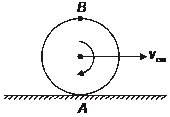# Disc Partitioned - 1

There is a circular disc of radius $R$ which is undergoing pure rolling on a surface.

If $V$ is the velocity of center of mass of the disc, then find the area of collection of points on the disc for which the magnitude of velocity is less than $V$ in SI units.

Given $R=1 \text{ meter}$.×